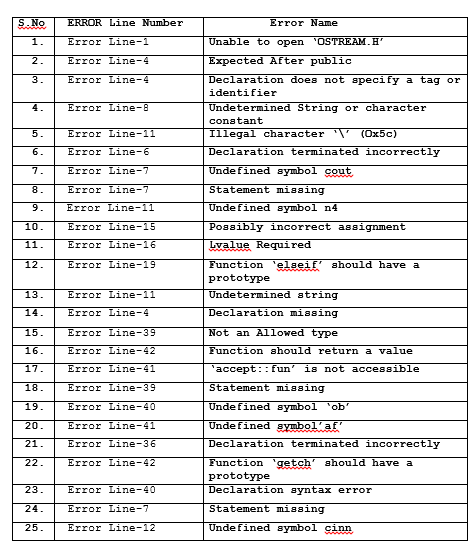# 25 most common errors while writing a C++ program

Today we are going to discuss most common errors while writing a C++ program.We have mentioned 25 errors in below program.

AIM-Write Any Program incorrectly and write all errors comes during execution?

#include<ostream.h>
#include<conio.h>
class accept
{ void fun)
{int n,a,b,sum,sub,multi,div,;
public:
cout<<“\n1.sum;
out<<“\n2.Subtract”
cout<<“\n3.Multiplication”;
cout<<\n4.Division”;
cinn;
cout<<“enter two number on which you want to done operation”; cin>>a>b;
if(n=1)
{a+b=sum;
cout<<“Sum=”<<sum;
}
Else if(n==2)
{sub=a-b;
cout<<“After Subtraction=”<<sub;
}
else if(n==3)
{multi=a*b;
cout<<“After multiplication=”multi;
}
else if(n==4)
{div=a/b;
cout<<“After Division=”<<div;
}
else2
cout<<“WRONG CHOICE”;
}
}
main()
{int 2
cout< clrscr();
accept ob
ob.fun();
af=420;
}